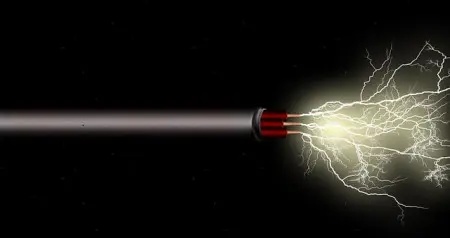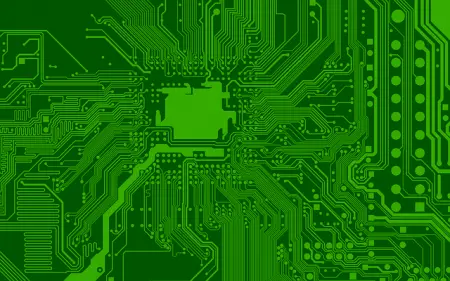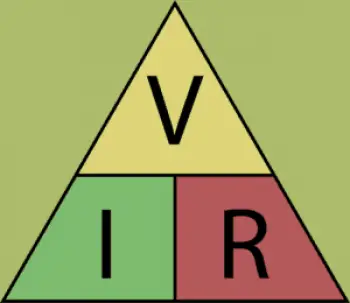# Basics of Electricity: Introduction and DefinitionsElectricity is an omnipresent force in our lives. From lighting our homes to powering electronic devices and keeping entire industries running, electricity is an essential element of modern society.

However, to most people, the concepts behind electricity can seem mysterious and complex.

In this section, we will explain the basics of electricity in an accessible and understandable way, allowing you to better appreciate how this fundamental phenomenon works.

## Electric Charge

Electricity originates in the fundamental particles of matter: electrons and protons. These particles have a property called "electric charge."

Electrons have a negative charge, while protons have the same charge but with a positive sign.

Electrons tend to be repelled by each other due to their equal charges, while electrons and protons tend to be attracted to each other due to their opposite charges.

## Electric CurrentElectric current is the flow of electrons through a conductor, such as a metal wire. This flow is initiated by a difference in electrical potential, commonly called "voltage."

Voltage is what drives the electrons to move in a particular direction along the conductor. The unit of measurement for electric current is the ampere (A).

### Types of Current: Direct and Alternating

There are two main types of electrical current: direct (DC) and alternating (AC).

• Direct current flows in a single constant direction, such as that provided by a battery or photovoltaic panel.

• Alternating current, on the other hand, changes direction periodically at a specific frequency, usually 50 or 60 times per second. This type of current is the dominant form of electricity in most power grids and is used in most of the electronic devices we use in our daily lives.

## Voltage

Voltage, represented by the letter "V" and measured in volts (V), is the driving force behind the flow of electrical current. Essentially, voltage represents the difference in electrical energy between two points in a circuit.

The greater the voltage difference between two points, the greater the force with which electrons will move from one point to another.

## Endurance

Electrical resistance, symbolized by the letter "R" and measured in ohms (Ω), is a property that opposes the flow of electric current.

Conductive materials, such as metals, generally have low resistance, allowing electrons to flow easily. On the other hand, insulating materials, such as rubber or plastic, have high resistance and hinder the flow of current.

## Electrical CircuitsAn electrical circuit is a closed path along which electrons can circulate.

The basic components of a circuit include a power source (such as a battery or generator), conductors (such as metal wires), and charging devices (such as light bulbs or appliances).

When a circuit is closed, electrons can flow from the power source through conductors to charging devices, doing work in the process.

## Electrical Power

Electrical power is the amount of electrical energy that is transferred or consumed per unit of time. Power is an important factor in determining the power consumption and capacity of electrical devices.

It is measured in watts (W) and is calculated using the formula P = V * I, where "P" is power, "V" is voltage, and "I" is current.

## Electricity Laws

The laws of electricity are fundamental principles that govern the behavior of electrical systems and circuits.The best known is Ohm's Law, which establishes the relationship between voltage (V), current (I) and resistance (R) in an electrical circuit. This law is essential to understanding how electric current flows in response to voltage and resistance in a circuit.

In addition to Ohm's Law, there are other specific laws in electricity that describe more particular phenomena, such as Gauss's law for static electricity or Coulomb's law for the force between electric charges.

In addition to the basic concepts of electricity mentioned so far, there are other more advanced concepts that delve into the complexities of electric current and its application in modern technology.

Some of the most important advanced concepts include:

• Frequency : Frequency refers to the number of cycles per second in an AC signal, measured in Hertz (Hz). It is a fundamental parameter in AC electricity and affects the way electrical devices work and interconnect.

• Impedance : Impedance is an electrical property that measures the resistance to current flow in AC circuits. It is made up of resistance, inductance and capacitance, and is essential in the design of electrical circuits and systems.

• Reactance : Reactance is a component of impedance in AC circuits that relates to the ability to store and release energy in the form of a magnetic or electric field.

• Power Electronics : This field focuses on the conversion and control of electrical energy. It includes devices such as rectifiers, inverters and motor controllers, which are vital in industrial and consumer electronics applications.

• Semiconductors : Semiconductors, like transistors, are the basis of modern electronics. These devices allow the amplification and control of electrical signals, and are essential in the manufacture of integrated circuits and microchips.

Author:

Published: September 28, 2023
Last review: September 28, 2023